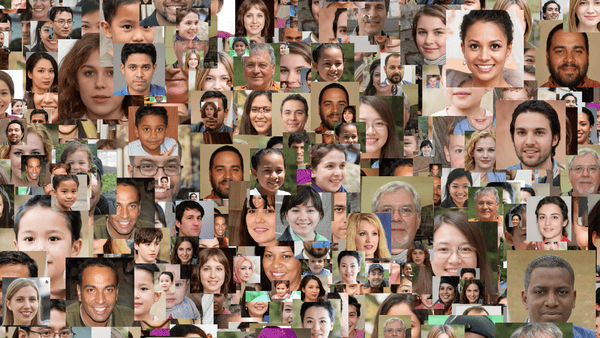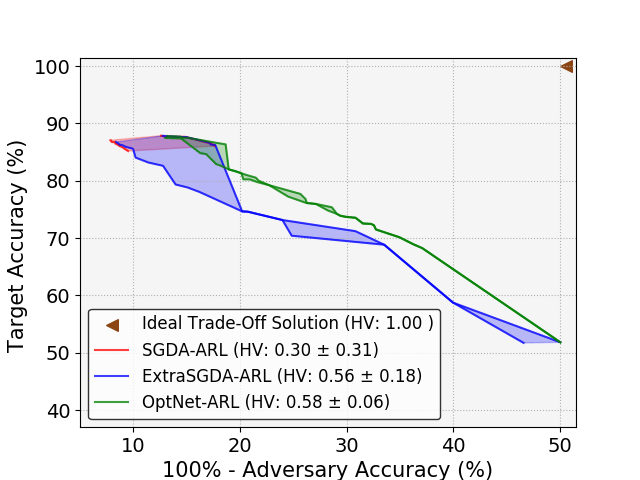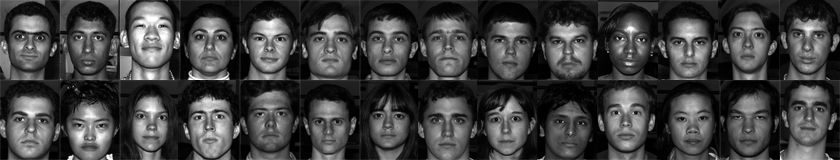Towards Fairness in Biometric Systems: Fundamental Trade-Offs and Algorithms

German Biometrics Working Group Meeting

September 14, 2020

VishnuBoddeti

## Progress In Biometrics

Face
Fingerprint
Iris/Periocular
Gait

Key Driver
Data, Compute, Algorithms

## State-of-Affairs

(report from the real-world)
"Tay, Microsoft's AI chatbot, gets a crash course in racism from Twitter""FaceApp's creator apologizes for the app's skin-lightening 'hot' filter""Facial recognition is accurate, if you're a white guy"• Boulamwini and Gebru, "Gender Shades:Intersectional Accuracy Disparities in Commercial Gender Classification," FAT 2018
"The Secretive Company That Might End Privacy as We Know It"Real world biometric recognition systems are effective but,

are biased,

violate user’s privacy and

not trustworthy.

## Today's Agenda

Build biometric systems that are fair and trustworthy.
Fair and Trustworthy ML

Mechanism: control semantic information in data representations

## 100 Years of Data RepresentationsControl Mathematical Concepts
variance, sparsity, translation, rotation, scale, etc.

## Bias in Learning

• Training:• Inference: Microsoft Gender classification• Boulamwini and Gebru, "Gender Shades:Intersectional Accuracy Disparities in Commercial Gender Classification," FAT 2018

## Privacy Leakage

• Training:• Inference: Microsoft Smile classification• B. Sadeghi, L. Wang, V.N. Boddeti, "Adversarial Representation Learning With Closed-Form Solvers," CVPRW 2020

## Information Leakage from Representations

• Learned Embeddings:• Attacks on Embeddings:
• Face reconstruction from template
• Mai et. al., ‘‘On the reconstruction of face images from deep face templates," IEEE Transactions on Pattern Analysis and Machine Intelligence, 2018
What is going on?
Dark Secret of Deep Learning

Recklessly absorb all statistical correlations in data

## Next Era of Biometric RepresentationsControl Semantic Concepts
age, gender, domain, etc.

## Controlling Semantic Information

• Target Concept: Smile & Private Concept: Gender
•• Problem Definition:
• Learn a representation $\mathbf{z} \in \mathbb{R}^d$ from data $\mathbf{x}$
• Retain information necessary to predict target attribute $\mathbf{t}\in\mathcal{T}$
• Remove information related to a desired sensitive attribute $\mathbf{s}\in\mathcal{S}$

## Technical Challenge

• How to explicitly control semantic information in learned representations?

• Can we explicitly control semantic information in learned representations?
The Can

Short Answer: Yes, we can, sometimes.

## A Subspace Geometry Perspective

• Case 1: when $\mathcal{S} \perp \!\!\! \perp \mathcal{T}$ (Gender, Age)• Case 3: when $\mathcal{S} \sim \mathcal{T}$ ($\mathcal{T}\subseteq\mathcal{S}$)• Case 2: when $\mathcal{S} \not\perp \!\!\! \perp \mathcal{T}$ (Car, Wheels)• B. Sadeghi, L. Wang, V.N. Boddeti, ‘‘Adversarial Representation Learning with Closed-Form Solutions," CVPRW 2020
The How

## A Fork in the Road

• Design metric to measure semantic attribute information
• not obvious how

• Learn metric to measure semantic attribute information
• probably feasible

## Game Theoretic Formulation• Three player game between:
• Encoder extracts features $\mathbf{z}$
• Target Predictor for desired task from features $\mathbf{z}$
• Adversary extracts sensitive information from features $\mathbf{z}$
\begin{equation} \begin{aligned} \min_{\mathbf{\Theta}_E,\mathbf{\Theta}_T} & \underbrace{\color{cyan}{J_t(\mathbf{\Theta}_E,\mathbf{\Theta}_T)}}_{\color{cyan}{\mbox{error of target}}} \quad s.t. \mbox{ } \min_{\mathbf{\Theta}_A} \underbrace{\color{orange}{J_s(\mathbf{\Theta}_E,\mathbf{\Theta}_A)}}_{\color{orange}{\mbox{error of adversary}}} \geq \alpha \nonumber \end{aligned} \end{equation}
• Adversary: learned measure of semantic attribute information

## How do we learn model parameters?• Update target while keeping encoder and adversary frozen.
• Update adversary while keeping encoder and target frozen.
• Update encoder while keeping target and adversary frozen.

## Three Player Game: Linear Case• Global solution is $(w_1, w_2, w_3)=(0, 0, 0)$
What we get

## Our Contributions

• Non-Zero Sum Formulation for Iterative Methods (CVPR'19)
• Standard setting, each player is a deep neural network.
• Local optima

• Global Optima for Kernel Methods (ICCV'19)
• Simplified setting, each player is linear.
• closed form solution + stable + performance bounds

• Hybrid Model with CNNs and Closed-Form Solvers (CVPRW'20)
• Standard setting, encoder is a deep neural network, other players are closed-form solvers.
• Local optima

## Optimizing Likelihood Can be Sub-Optimal

Encoder
• Limitations:
• Encoder target distribution leaks information !!
• Practice: simultaneous SGD does not reach equilibrium
• Class Imbalance: likelihood biases solution to majority class

## Maximum Entropy Adversarial Representation Learning

Encoder

Converges to Local Optima

## Maximum Entropy ARL Continued...• Three player game between:
• Encoder extracts features $\mathbf{z}$
• Target Predictor for desired task from features $\mathbf{z}$
• Adversary extracts sensitive information from features $\mathbf{z}$
• Three Player Non-Zero Sum Game:
• \begin{equation} \begin{aligned} \min_{\mathbf{\theta}_A} & \mbox{ } \underbrace{\color{orange}{J_1(\mathbf{\theta}_E,\mathbf{\theta}_A)}}_{\color{orange}{\mbox{error of adversary}}} \\ \min_{\mathbf{\theta}_E,\mathbf{\theta}_T} & \mbox{ } \underbrace{\color{cyan}{J_2(\mathbf{\theta}_E,\mathbf{\theta}_T)}}_{\color{cyan}{\mbox{error of target}}} - \alpha \underbrace{\color{orange}{J_3(\mathbf{\theta}_E,\mathbf{\theta}_A)}}_{\color{orange}{\mbox{entropy of adversary}}} \nonumber \end{aligned} \end{equation}

## Geometry of Optimization

\begin{equation} \begin{aligned} \min_{\mathbf{\Theta}_E} & \ \ {\color{cyan}{J_t(\mathbf{\Theta}_E)}} \\ \mathrm {s.t. \ \ } & {\color{orange}{J_s (\mathbf{\Theta}_E) \ge \alpha}} \nonumber \end{aligned} \end{equation}• Non-convexity: feasible set is non-convex
• Non-differentiability: solution is either a plane or a line
B. Sadeghi, R. Yu, V.N. Boddeti, ‘‘On the Global Optima of Kernelized Adversarial Representation Learning," ICCV 2019

## Solution: Spectral Adversarial Representation Learning

• Lagrangian formulation:
• \begin{equation} \min_{\mathbf{\Theta}_E} \Big\{(1-\lambda){\color{cyan}{J_t(\mathbf{\Theta}_E)}}- (\lambda) {\color{orange}{J_s (\mathbf{\Theta}_E)} }\Big\} \nonumber \end{equation}

Non-Convex + Non-Differentiable

• Solution:
• \begin{equation} \mathbf{\Theta}_E, r^*=\mbox{Negative Eig} \Big\{\mathbf{X}\left(\lambda \color{orange}{\mathbf{S}^T \mathbf{S}} - (1-\lambda)\color{cyan}{\mathbf{Y}^T \mathbf{Y}} \right)\mathbf{X}^T \Big\}\nonumber \end{equation}

Global Optima + Optimal Dimensionality + Performance Bounds

B. Sadeghi, R. Yu, V.N. Boddeti, "On the Global Optima of Kernelized Adversarial Representation Learning," ICCV 2019

## Closed-Form Solvers• Encoder extracts features $\mathbf{z}$
• Target Predictor: kernel ridge regressor to predict target from $\mathbf{z}$
• Adversary: kernel ridge regressor to extract sensitive information from $\mathbf{z}$
B. Sadeghi, L. Wang, V.N. Boddeti, "Adversarial Representation Learning with Closed-Form Solutions," CVPRW 2020

## Properties of Ideal Embedding• Embedding Dimensionality
• # of negative eigenvalues of
• \begin{equation} \mathbf{B} = \lambda \tilde{\mathbf{S}}^T \tilde{\mathbf{S}} -(1-\lambda)\tilde{\mathbf{Y}}^T \tilde{\mathbf{Y}} \end{equation}

## Application-1: Fair Classification

• UCI Adult Dataset (creditworthiness, gender)
Method Income Gender $\Delta^*$
Raw Data 84.3 98.2 22.8
Remove Gender 84.2 83.6 16.1
Zero-Sum game 84.4 67.7 0.3
Non-Zero-Sum Game 84.6 67.3 0.1
Global-Optima 84.1 67.4 0.0
Hybrid 83.8 67.4 0.0
$^*$ Absolute difference between adversary accuracy and random chance

## Application-2: Mitigating Privacy Leakage• CelebA Dataset (smile, gender)
Method Smile Gender $\Delta^*$
Raw Data 93.1 82.9 21.5
Zero-Sum game 91.8 72.5 11.1
Non-Zero-Sum Game 91.6 62.1 0.7
Global-Optima 92.0 61.4 0.0
Hybrid 92.5 61.4 0.0
$^*$ Absolute difference between adversary accuracy and random chance

## Application-3: Mitigating Privacy Leakage## Application-4: Illumination Invariance• 38 identities and 5 illumination directions
• Target:Identity Label
• Sensitive:Illumination Label

## Open Questions

• Understand fundamental trade-off between utility and fairness.
• Understand achievable trade-off between utility and fairness.
• Optimization of adversarial training, especially three player games under general settings.
• $\dots$

## Summary

• Striving step towards explicit control of,
• semantic information in learned representations